# Fully Configured Transmission Line

Figure C.4 illustrates a three-way combination of source impedance, transmission line, and load impedance.

Figure C.4. A transmission line complete with source and load impedances may be modeled as a cascade of three two-port circuits.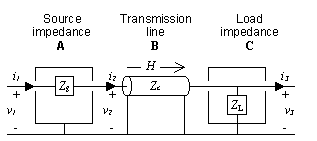The input impedance of the loaded transmission line may be determined from inspection of the cascaded combination of BC . This part of the system represents the transmission line and its load.

Equation C.9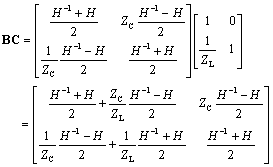The input impedance v 2 / i 2 equals the ratio BC 0,0 / BC 1,0 .

Equation C.10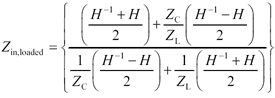Multiplying both numerator and denominator by the factor Z C simplifies the structure of the fraction somewhat. For now, leave the sum-and-differences of the H terms unmolested, as you will have an opportunity to develop some interesting approximations for these terms later.

Equation C.11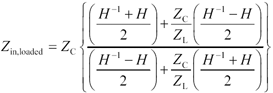Some interesting simplifications can be teased out of [C.11] under special conditions. When Z L is very large, the left-hand terms in the numerator and denominator of [C.11] dominate. When Z L / Z C = 1, the numerator and denominator exactly cancel. When Z L is very small, only the right-hand terms matter.

Equation C.12Equation C.13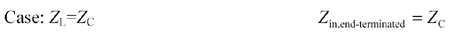Equation C.14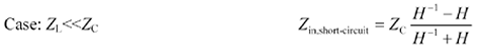The gain (voltage transfer function v 3 / v 1 ) of the loaded transmission line may be determined from inspection of the cascaded combination of all three parts ABC . This matrix represents the combination of source, transmission line, and load.

Equation C.15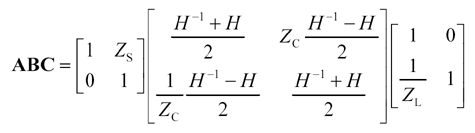In phasor notation, the voltage gain G FWD = v 3 / v 1 equals the inverse of the first element of ABC .

Equation C.16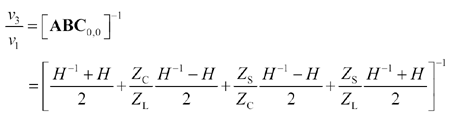The voltage gain expression may be simplified somewhat by factoring related terms.

Equation C.17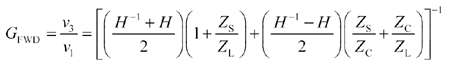The response measured by a time-domain reflectometer (TDR) would be the gain from v 1 to v 2 . You can compute G TDR = v 2 / v 1 as the product of v 3 / v 1 , which is given by [C.17], times the upper-left member of matrix BC , which represents the ratio v 2 / v 3 under the condition i 3 = 0.

Equation C.18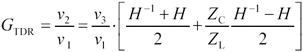In expanded form,

Equation C.19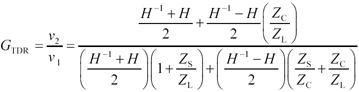High-Speed Signal Propagation[c] Advanced Black Magic
ISBN: 013084408X
EAN: N/A
Year: 2005
Pages: 163